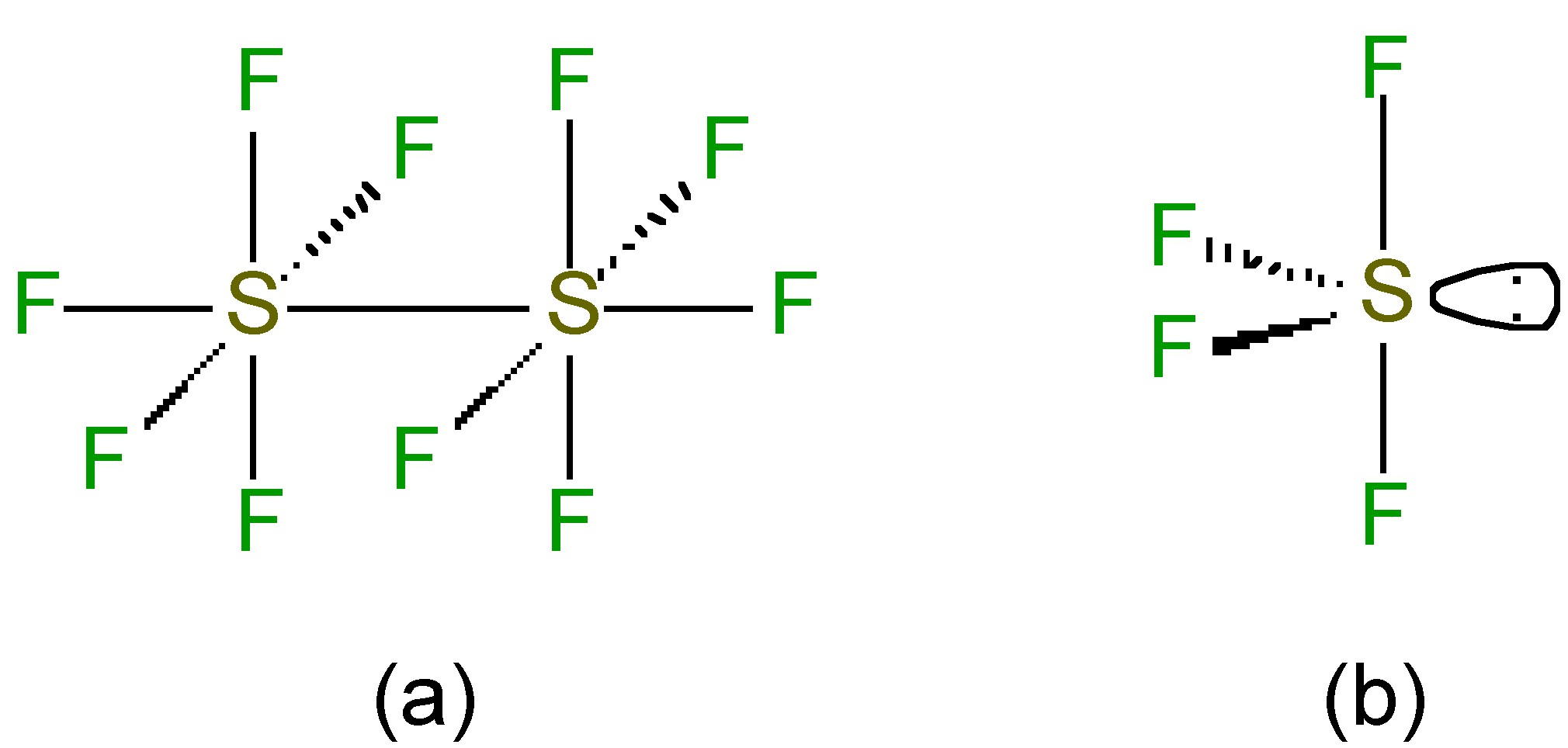# 9.9: Sulfur Halides

•• Contributed by Andrew R. Barron
• Professor (Chemistry) at Rice University
• Sourced from CNX

## Sulfur hexafluoride

Sulfur hexafluoride (SF6) is a gas at standard temperature and pressure (25 °C, 1 atm). The most common synthesis involves the direct reaction of sulfur with fluorine yields SF6.

$\rm S + 3 F_2 \rightarrow SF_6$

It should be noted that while SF6 is highly stable, SCl6 is not formed. The explanation of this difference may be explained by a consideration of the Born-Haber cycle shown in Figure $$\PageIndex{1}$$. A similar cycle may be calculated for SCl6; however, a combination of a higher dissociation energy for Cl2 and a lower S-Cl bond energy (Table $$\PageIndex{1}$$) provide the rational for why SCl6 is not formed.Figure $$\PageIndex{1}$$: Born-Haber cycle for the formation (ΔHf) of SF6: where D(X-Y) = dissociation energy for X-Y bond, E(S-F) = S-F bond energy, and S* indicates 6 coordinate sulfur.
 Bond dissociation energy kJ/mol Bond energy kJ/mol D(F-F) 158 E(S-F) 362 D(Cl-Cl) 262 E(S-Cl) 235

The S-F bond length (1.56 Å) is very short and consistent with π-bonding in addition to σ-bonding. Like SiF62-, SF6 is an example of a hypervalent molecule (Figure $$\PageIndex{2}$$).Figure $$\PageIndex{2}$$: Molecular orbital bonding in SF6.

Sulfur hexafluoride is an unreactive, non toxic compound. Its inert nature provides one of its applications, as a spark suppressor. The hexafluoride is generally resistant to chemical attack, e.g., no reaction is observed with potassium hydroxide (KOH) at 500 °C. The low reactivity is due to SF6 being kinetically inert due to:

• Coordination saturation precluding associative reactions with nucleophiles.
• Strong S-F bond (360 kJ/mol) limiting dissociative reactions.

Thermodynamically SF6 should react with water (ΔH = -460 kJ/mol), but the rate factors are too great. Sulfur hexafluoride can be reduced with sodium in liquid ammonia, (9.9.2), or with LiAlH4. In each of these reactions the mechanism involves the formation of a radical, (9.9.3). The reaction with sulfur trioxide yields SO2F2, (9.9.4), however, the reactions with carbon or CS2 only occur at elevated temperatures (500 °C) and pressure (4000 atm).

$\rm SF_6 + 8 Na \rightarrow Na_2S + 6 NaF$

$\rm SF_6 + e^- \rightarrow SF_6^-$

$\rm SF_6 + 2 SO_3 \xrightarrow{\text{250 °C}} 3 SO_2F_2$

## Sulfur monochloride pentafluoride

Although the hexachloride is unknown, it is possible to isolate the monochloride derivative (SF5Cl) by the oxidative addition of Cl-F across SF4.

$\rm SF_4 + ClF \rightarrow SF_5Cl$

Sulfur monochloride pentafluoride is a gas (Bp = -21 °C), but unlike SF6 it is fairly reactive due to the polarization of the S-Cl bond (Figure $$\PageIndex{3}$$), and as a consequence it reacts with water, (9.9.6).

$\rm SF_5Cl + 3 H_2 \rightarrow SO_3 + 5 HF + HCl$Figure $$\PageIndex{3}$$: Polarization of the S-Cl bond in SF5Cl.

## Sulfur pentafluoride

Although SF5 does not exist as a stable molecule, the gaseous dimmer S2F10 (Bp = 29 °C) may be isolated from the photochemical hydrogen reduction of SF5Cl, (9.9.7).

$\rm 2 SF_5Cl + H_2 \xrightarrow{h\nu} S_2F_{10} + 2 HCl$

While the sulfur is octahedral in S2F10 (Figure $$\PageIndex{4}$$a) the S-S bond is weak and long (2.21 Å versus an expected 2.08 Å for a single S-S bond). Despite the apparently weak S-S bond, S2F10 shows almost no reactivity at room temperature; however, the S-S bond undergoes homoleptic cleavage at high temperatures. The resultant SF5. radicals disproportionate to give highly reactive fluoride radicals, (9.9.8), which is the source of the highly oxidative properties of S2F10.

$\rm 2 SF_5 \rightarrow 2 SF_4 + 2 F\cdot$Figure $$\PageIndex{4}$$: Structures of (a) S2F10 and (b) SF4.

The SF5. fragment is stabilized by the addition of an alkyl radical, and thus, there are a large number of RSF5 derivatives known. Unlike, the chloride analog, these are very stable.

## Sulfur tetrafluoride

Sulfur tetrafluoride (SF4) is prepared from sulfur dichloride and sodium fluoride in acetonitrile solution at 70 - 80 °C.

$\rm 3 SCl_2 + 4 NaF \rightarrow SF_4 + S_2Cl_2 + 4 NaCl$

The structure of SF4 (and its substituted derivatives RSF3) is based upon a trigonal bipyramidal structure with one of the equatorial sites being occupied by a lone pair (Figure $$\PageIndex{4}$$b). Unlike the hexafluoride, sulfur tetrachloride is a highly reactive compound. It hydrolyzes readily, (9.9.10), and is a useful fluorinating agent (Figure $$\PageIndex{5}$$).

$\rm SF_4 + 2 H_2O \rightarrow SO_2 + 4 HF$Figure $$\PageIndex{5}$$: Examples of the use of SF4 as a fluorinating agent.

## Sulfur chlorides

The chlorination of molten sulfur yields the fowl smelling disulfur dichloride (S2Cl2). If the reaction is carried out with a catalyst such as FeCl3, SnI4 or I2, an equilibrium mixture containing sulfur dichloride (SCl2) is formed. However, the dichloride dissociates readily, (9.9.11), although it can be isolated as a dark red liquid if it distilled in the presence of PCl5. The reaction of chlorine at -80 °C with SCl2 or S2Cl2 allows for the formation of SCl4 as a yellow crystalline compound which dissociates above -31 °C. Sulfur chlorides are readily hydrolyzed. Sulfur chlorides are used to dissolve sulfur (giving species up to S100Cl2) for the vulcanization of rubber.

$\rm 2 SCl_2 \rightleftharpoons S_2Cl_2 + Cl_2$

In the vapor phase S2Cl2 has C2 symmetry (Figure $$\PageIndex{6}$$a) while that of SCl2 has C2v symmetry (Figure $$\PageIndex{6}$$b).Figure $$\PageIndex{6}$$: Structures of (a) S2Cl2 and (b) SCl2.